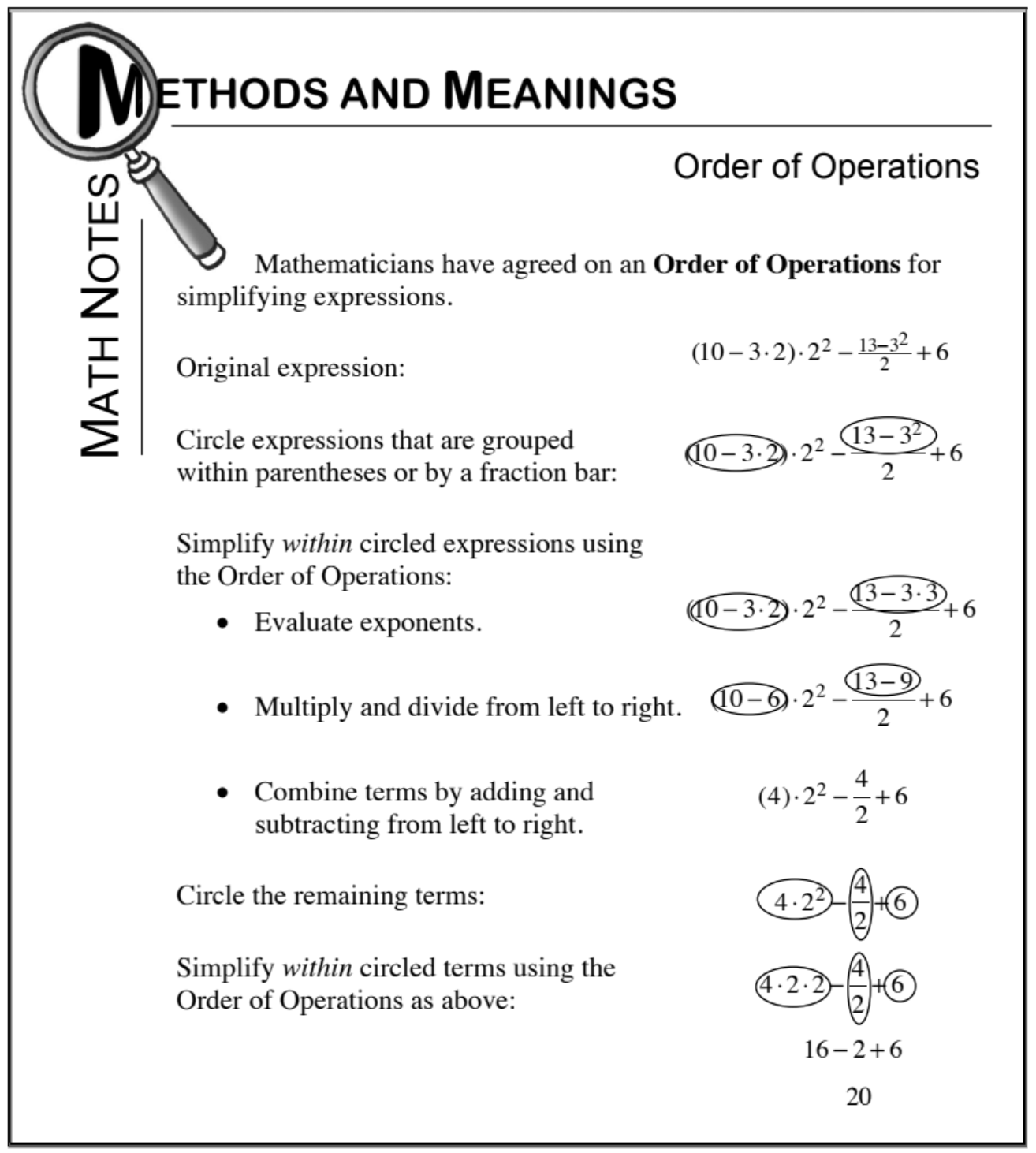### Home > CC1 > Chapter 7 > Lesson 7.1.2 > Problem7-22

7-22.

Simplify the expressions below. Homework Help ✎

For parts (a) through (d), refer to the Math Notes box from Lesson 6.2.1 at the bottom of the page.

1. $4·4+4(5−2)+7$

Remember to calculate the value of each term before adding!

$35$
Be sure to show your work!

1. $7−9\div9+4(4−3)−7$

The expression in parentheses should be simplified first.
Then remember to always multiply and divide before adding and subtracting.

1. $−12+3(7−4)+5$

Try circling the terms before simplifying the expression.
That way you will be less likely to make a mistake!

Be careful adding and subtracting negative integers!

1. $3(15−2·6)−5$

Make sure you simplify the expression in parentheses first!

4
Don't forget to keep track of those negative integers!